Ex 7.5

Chapter 7 Class 12 Integrals
Serial order wise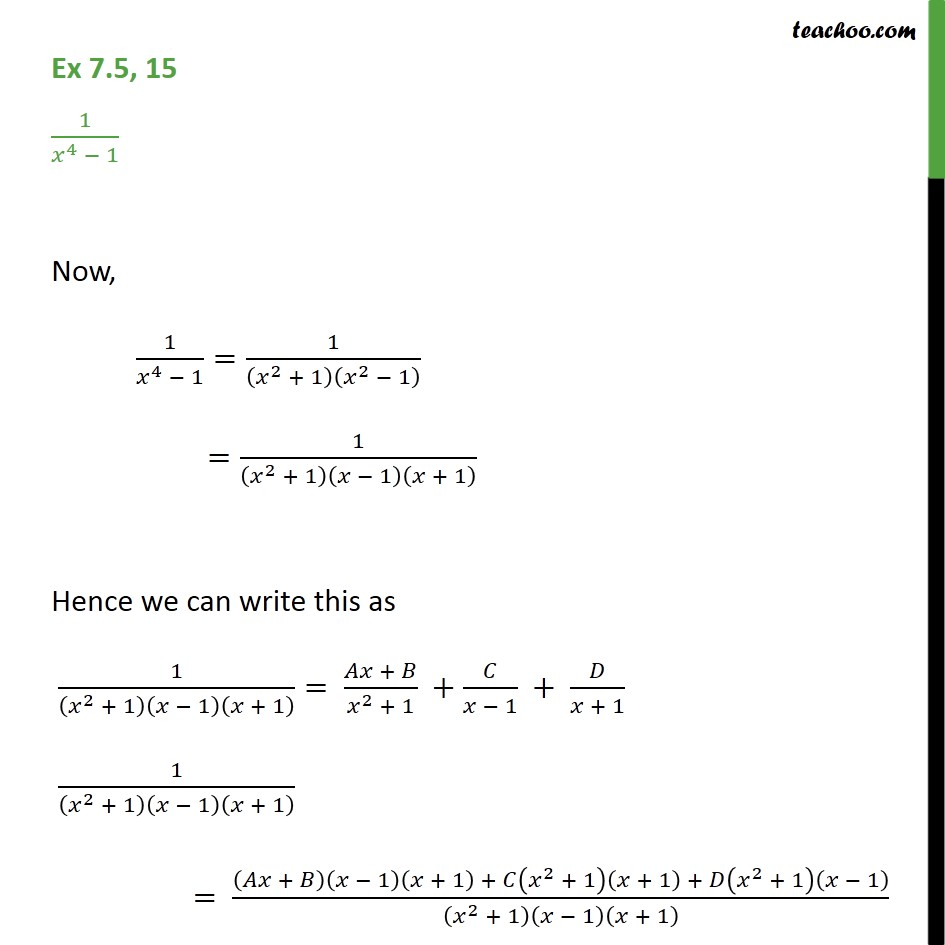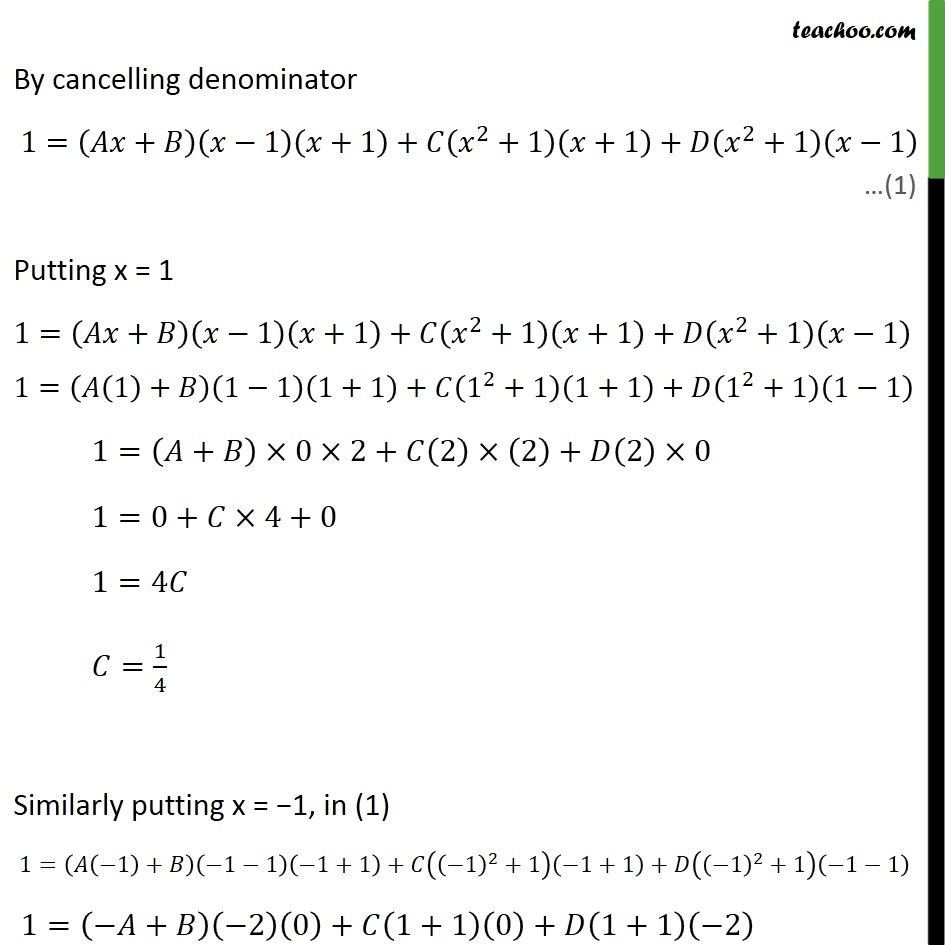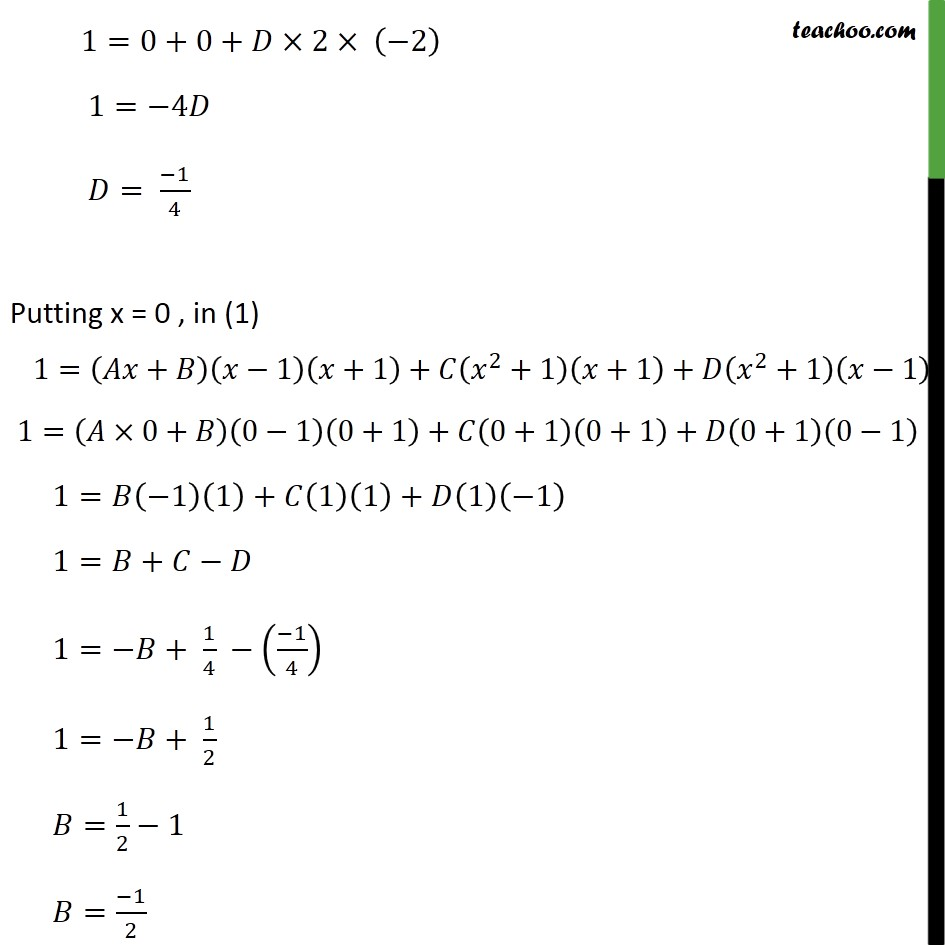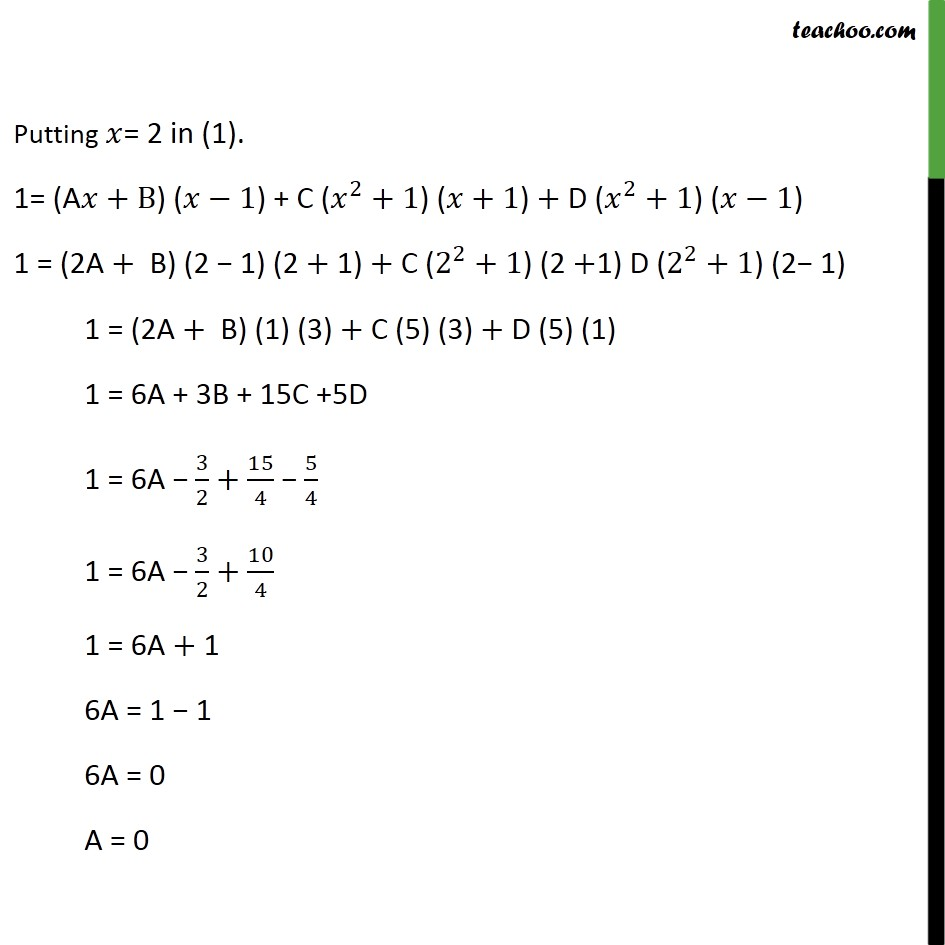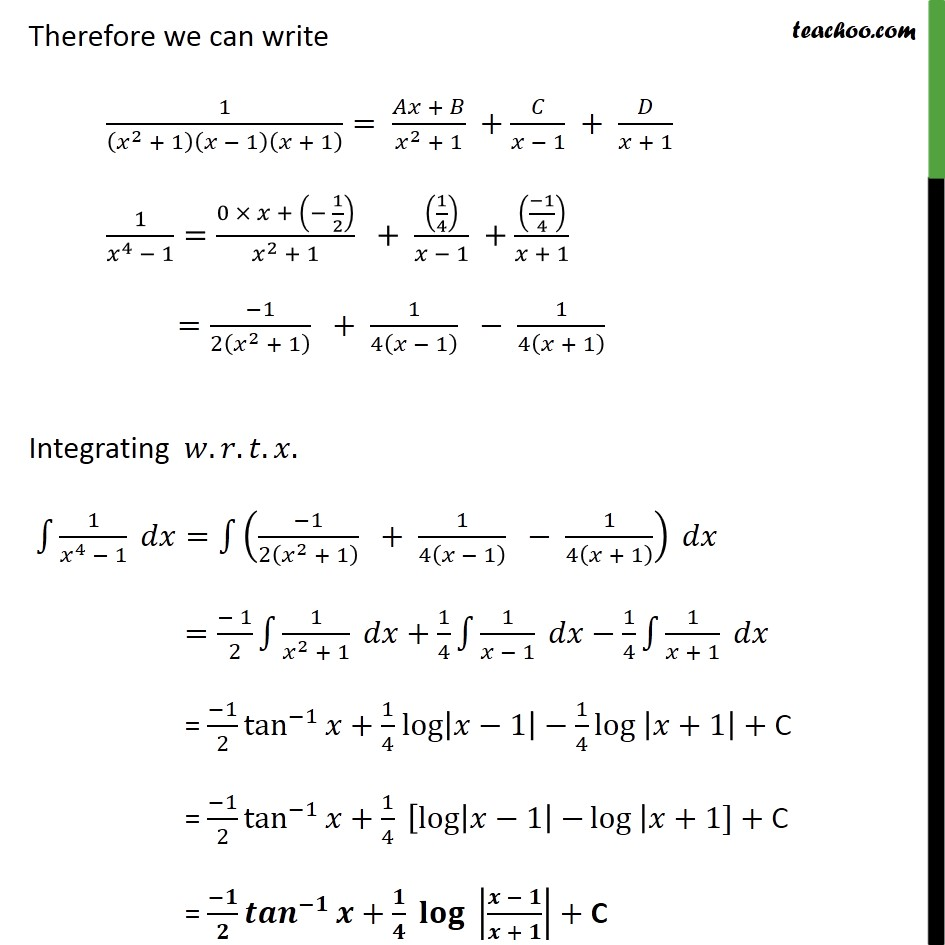Learn in your speed, with individual attention - Teachoo Maths 1-on-1 Class

### Transcript

Ex 7.5, 15 1 4 1 Now, 1 4 1 = 1 2 + 1 2 1 = 1 2 + 1 1 + 1 Hence we can write this as 1 2 + 1 1 + 1 = + 2 + 1 + 1 + + 1 1 2 + 1 1 + 1 = + 1 + 1 + 2 + 1 + 1 + 2 + 1 1 2 + 1 1 + 1 By cancelling denominator 1= + 1 +1 + 2 +1 +1 + 2 +1 1 Putting x = 1 1= + 1 +1 + 2 +1 +1 + 2 +1 1 1= 1 + 1 1 1+1 + 1 2 +1 1+1 + 1 2 +1 1 1 1= + 0 2+ 2 2 + 2 0 1=0+ 4+0 1=4 = 1 4 Similarly putting x = 1, in (1) 1= 1 + 1 1 1+1 + 1 2 +1 1+1 + 1 2 +1 1 1 1= + 2 0 + 1+1 0 + 1+1 2 1=0+0+ 2 2 1= 4 = 1 4 Putting x = 0 , in (1) 1= + 1 +1 + 2 +1 +1 + 2 +1 1 1= 0+ 0 1 0+1 + 0+1 0+1 + 0+1 0 1 1= 1 1 + 1 1 + 1 1 1= + 1= + 1 4 1 4 1= + 1 2 = 1 2 1 = 1 2 Putting = 2 in (1). 1= (A +B) ( 1) + C ( 2 +1) ( +1) + D ( 2 +1) ( 1) 1 = (2A + B) (2 1) (2 + 1) + C ( 2 2 +1) (2 +1) D ( 2 2 +1) (2 1) 1 = (2A + B) (1) (3) + C (5) (3) + D (5) (1) 1 = 6A + 3B + 15C +5D 1 = 6A 3 2 + 15 4 5 4 1 = 6A 3 2 + 10 4 1 = 6A + 1 6A = 1 1 6A = 0 A = 0 Therefore we can write 1 2 + 1 1 + 1 = + 2 + 1 + 1 + + 1 1 4 1 = 0 + 1 2 2 + 1 + 1 4 1 + 1 4 + 1 = 1 2 2 + 1 + 1 4 1 1 4 + 1 Integrating . . . . 1 4 1 = 1 2 2 + 1 + 1 4 1 1 4 + 1 = 1 2 1 2 + 1 + 1 4 1 1 1 4 1 + 1 = 1 2 tan 1 + 1 4 log 1 1 4 log +1 + C = 1 2 tan 1 + 1 4 log 1 log +1]+ C = + + + C